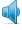Graph - definition, pronunciation, transcription

Amer.  |ɡræf|Brit.  |ɡrɑːf|noun

- a visual representation of the relations between certain quantities, represented as points, plotted with reference to a set of axes
- a visual display of information (syn: chart)
- a written symbol that is used to represent speech (syn: character, grapheme)

verb

- represent by means of a graph (syn: chart)
- plot upon a graph

Examples

Students were asked to graph each equation.

Martin showed me a graph of their recent sales.

The computer can draw the graph for you.

The graph shows that murders in the city declined from a peak of 173 in 2004.

Students plotted soil temperatures on a graph throughout the school year.

The shaded part of the graph represents the amount of sales.

the vertical axis of a graph

Line the pages for the graph both horizontally and vertically.

Keith was drawing a complicated-looking graph.

Sales targets are indicated on the graph by a vertical dotted line.

We plotted a graph to show the increase in sales figures this year.

From this graph, it can be seen that some people are more susceptible to the disease.

The graph showed peaks and troughs of activity.

we will refer to the plane of the graph as the X-Y plane

noun
singular: graph
plural: graphs